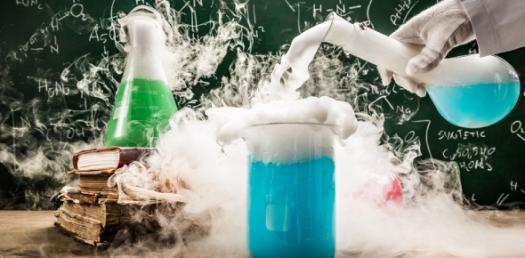# Hs Chemistry Practice Final

50 Questions | Attempts: 3079
ShareSettingsPractice final exam for the first semester of basic high school chemistry.

• 1.
Convert 57.03mm to meters
• A.

0.005703m

• B.

0.05703m

• C.

0.5703m

• D.

57,030.0m

• 2.
Convert 17.2°C to Kelvin
• A.

256.35 K

• B.

298.15 K

• C.

237.35 K

• D.

290.35 K

• 3.
Convert 0.00924kg to mg
• A.

9.240mg

• B.

92.40mg

• C.

924.0mg

• D.

9240mg

• 4.
Convert 375.12 K to °C
• A.

275.12°C

• B.

648.27°C

• C.

101.97°C

• D.

475.12°C

• 5.
You have a 25mL graduated cylinder and an unknown mass of Zinc. You fill the graduated cylinder with 10mL of water and drop the sample of Zinc in carefully. The water rises to 12mL. If the density of Zinc is 7.14 g/mL, what is the mass of the sample of Zinc used?
• A.

0.280g

• B.

14.3g

• C.

92.8g

• D.

107g

• 6.
Which of the following elements are transition metals? (You may select multiple answers)
• A.

Manganese (Mn)

• B.

Silicon (Si)

• C.

Strontium (Sr)

• D.

Gold (Au)

• 7.
Which of the following elements has the largest atomic radius?
• A.

Nitrogen (N)

• B.

Zinc (Zn)

• C.

Tungsten (W)

• D.

• 8.
Order the following elements in order of increasing ionization energy: Hg, Fr, Cl, F, As
• A.

F, Cl, As, Hg, Fr

• B.

Fr, Hg, As, Cl, F

• C.

As, F, Cl, Fr, Hg

• D.

Hg, Fr, Cl, F, As

• 9.
True or False: Lithium (Li) is an alkaline earth metal.
• A.

True

• B.

False

• 10.
Which of the following elements are Lanthanoids? (You may select multiple answers)
• A.

• B.

Einsteinium (Es)

• C.

Potassium (K)

• D.

Terbium (Tb)

• 11.
Which element has a mass number of 32?
• A.

Sulfur (S)

• B.

Germanium (Ge)

• C.

Chlorine (Cl)

• D.

Arsenic (As)

• 12.
How many neutrons are present in a Carbon-14 atom?
• A.

4 neutrons

• B.

6 neutrons

• C.

8 neutrons

• D.

10 neutrons

• 13.
An atom of which element has 12 protons, 12 neutrons, and 12 electrons?
• A.

Carbon (C)

• B.

Nitrogen (N)

• C.

Magnesium (Mg)

• D.

Sodium (Na)

• 14.
What is the name of an atom with an atomic mass number of 18 and 8 protons?
• A.

Oxygen-8

• B.

Oxygen-16

• C.

Oxygen-18

• D.

Oxygen-26

• 15.
True or False: Calcium (Ca) always has 20 protons.
• A.

True

• B.

False

• 16.
True or False: Na+ is an anion.
• A.

True

• B.

False

• 17.
What is the name for the ion CO32- ?
• A.

Carbon Trioxide

• B.

Carbonoxide

• C.

Carbonate

• 18.
What is the formula for Aluminum (III) Sulfate?
• A.

Al3SO4

• B.

Al3S

• C.

Al2(SO4)3

• D.

Al2S3

• 19.
What is the name for Cu(NO3)2?
• A.

Copper Nitride

• B.

Copper Trinitrate

• C.

Copper (I) Nitrate

• D.

Copper (II) Nitrate

• 20.
Is OH- an element, ion, or compound?
• A.

Element

• B.

Ion

• C.

Compound

• 21.
What term describes the process of a substance changing from solid to gas?
• A.

Condensation

• B.

Deposition

• C.

Sublimation

• D.

Evaporation

• 22.
Which of the following is a chemical change?
• A.

Glass breaking

• B.

• C.

A towel absorbing water

• D.

A nail rusting in the rain

• 23.
True or False: An example of qualitative data is color.
• A.

True

• B.

False

• 24.
Which of the following characteristics of a person are quantitative? (You may select multiple answers)
• A.

Hair color

• B.

Height

• C.

Skin tone

• D.

Weight

• E.

Age

• 25.
Which of the following describes a heterogeneous substance containing large solid particles?
• A.

Compound

• B.

Colloid

• C.

Suspension

• D.

Solution

## Related TopicsBack to top
×

Wait!
Here's an interesting quiz for you.